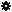﻿ Object Model: PolygonMesh.Set

PolygonMesh.Set

## PolygonMesh.Set### Description

Sets from a complete data description of the polygon mesh. If you use it on an object with some clusters and you change the topology the burden of updating the clusters is on the user.

Note: This method uses output arguments. C# and some scripting languages (such as JScript and PerlScript) don't support arguments passed by reference so you need to use the best workaround for your situation:

For scripting languages this method returns an ISIVTCollection which you can use to get the output arguments.

For C# the only available workaround is to create a VBScript custom command which returns both the output arguments and the return value in one array. For details, see What Happens when the Function Already Returns a Value?.

### C# Syntax

 ```PolygonMesh.Set( Object in_vertices, Object in_polygonData ); ```

### Scripting Syntax

 ```PolygonMesh.Set( [Vertices], [PolygonData] ); ```

### Parameters

Parameter Type Description
Vertices Array Array representing the polygon vertices. The array is a 2D array (Nx3) of x,y,z values.
PolygonData Array An ordered array of polygon definitions, each polygon is defined by a list of elements, the first element of a polygon definition must be set with the number of indices for that polygon. The ordering of vertices must respect a ccw ordering to get out going normals (right-hand rule). E.g. array of polygons with 4 indices each e.g. {4,0,1,4,3,4,1,2,5,4... }

### Examples

#### JScript Example

 ```// // This example shows how to get the polygonmesh description from // one geometry and apply it on another. // var oRoot = Application.ActiveSceneRoot; var oCube = oRoot.AddGeometry( "Cube","MeshSurface" ); var oCone = oRoot.AddGeometry( "Cone","MeshSurface" ); // convert VB array to JScript array var vbArgs = new VBArray(oCube.ActivePrimitive.Geometry.Get2()); var args = vbArgs.toArray(); // get the vertices var vbArg0 = new VBArray(args); var vertices = vbArg0.toArray(); // get the polygon information var vbArg1 = new VBArray(args); var polygonData = vbArg1.toArray(); // Freezing the cone operator stack. Otherwise the method will return // the error E_ACCESSDENIED. This was done in order to prevent writing transient // data to objects. Otherwise setting values on non Application.FreezeObj(oCone); // Converting the cone to a cube oCone.ActivePrimitive.Geometry.Set(vertices,polygonData); ```